# PSEB 7th Class Science Solutions Chapter 15 Light

## PSEB Solutions for Class 7 Science Chapter 15 Light

Science Guide for Class 7 PSEB Light Intext Questions and Answers

Think and Answer (Textbook Page No. 186)

Question 1.
The process of change in direction of light after falling on a mirror is called ……………….. of light.
Reflection.

Question 2.
Angle of incidence is equal to angle of reflection. (True/False)
True.Think and Answer (Textbook Page No. 189)

Question 1.
The distance between the object and the mirror is ……………… to the distance
between the image and the mirror.
Equal.

Question 2.
Thes plane mirror has to be placed vertically on the graph paper. (True/False)
True.

Question 3.
The plane mirror should be vertically upright.
Right.

Think and Answer (Textbook Page No. 191)

Question 1.
The image of the sun formed by the concave mirror on the wall is …………………… image.
(Real/Virtual)
Real.

Question 2.
Is the image of a candle seen using a concave mirror same as that the image of the Sun seen on the wall ?
In both cases the rays of light are reflected from a concave mirror to form a real image.

Think and Answer (Textbook Page No. 192)

Question 1.
When the object is very far from the concave mirror, the image formed is ………………….
and
Real, inverted/small in size.

Question 2.
The image formed on the screen is real.
True. (True/False)

Question 3.
For a concave mirror, the image formed is always real.
False.Think and Answer (Textbook Page No. 195) (True/False)

Question 1.
The bright spot of light obtained on the paper is the image formed due to effect.
Converging.

Question 2.
The image obtained on the paper is the virtual image.
False.

Think and Answer (Textbook Page No. 196)

Question 1.
The convex lens is placed between the object and the screen. (True/False)
True.

Question 2.
For a convex lens, the image formed is always real. (True/False)
False.

Think and Answer (Textbook Page No. 198)

Question 1.
White light is composed of ………………. Colours. (True/False)
Seven.

Question 2.
When disc with seven colours is rotated, it appears to be red. (True/False)
False.

Question 3.
Name the seven colours of which the white light is made of.
The seven colours of white light are:

1. Violets,
2. Indigo,
3. Blue,
4. Green,
5. Yellow,
6. Orange
7. Red.

Collectively called VIBGYOR.PSEB 7th Class Science Guide Light Textbook Questions and Answers

1. Fill in the Blanks:

(i) An image formed by a ………………….. mirror is always of the same size as that of object.
plane

(ii) In a plane mirror, the left hand of a person appears to be the ………………… hand in the image and ……………. hand appears to be the left hand in the image.
right, right

(iii) The image formed by a convex mirror is always ………………… and ……………….. in size.
erect, smaller

(iv) Convex lenses are ………………… in the middle and concave lenses are ……………….. in the middle than at the edges.
thin, thick

(v) A prism splits the white light into ……………….. colours.
seven

2. State True or False:

(i) There is reflection of light through a lens.
False

(ii) The ray of light coming towards the plane mirror is called the reflected ray.
False(iii) The image formed by a plane mirror is always in front of the mirror.
False

(iv) A concave mirror is a part of hollow sphere of glass, whose outer side is coated with silver layer and reflection takes place from the inside.
True

(v) Concave lens always forms a virtual, erect and smaller image of the object.
True

Question (i)
Which of the following does not show reflection of light ?
(a) Plane mirror
(b) Concave mirror
(c) Convex mirror
(d) Card board.
(d) Card board.

Question (ii)
Which is used for rear view in cars and other vehicles:
(a) Concave mirror
(b) Convex mirror
(c) Convex lens
(d) Concave lens.
(b) Convex mirror.

Question (iii)
The image of an object formed by a concave lens is always ?
(a) Real and diminished
(b) Virtual and larger
(c) Real and larger
(d) Virtual and diminished.
(d) Virtual and diminished.

Question (iv)
The process of splitting of white light in seven colours on passing through a prism is called:
(a) Reflection of light
(b) Refraction of light
(c) Bending of light
(d) Dispersion of light.
(d) Dispersion of light.4. Match the Column ‘I’ with Column ‘II’:

 Column ‘I’ Column ‘II’ 1. Mirror used by dentists (a) Spectacles 2. Rear view Mirror (b) Microscope 3. Magnifying glass (c) Vehicles 4. Concave Lens (d) Concave

 Column ‘I’ Column ‘II’ 1. Mirror used by dentists (d) Concave 2. Rear view Mirror (c) Vehicles 3. Magnifying glass (b) Microscope 4. Concave Lens (a) Spectacles.

5. Very Short Answer Type Questions:

Question (i)
Is the image formed by a plane mirror real or virtual ?
The image formed by the plane mirror is Virtual.

Question (ii)
Which lens forms a real image of an object ?
Convex lens forms a real image of the object.

Question (iii)
Which optical instrument uses convex lens ?
Convex lens is used in a microscope.

Question (iv)
What is the seven rainbow coloured disc called ?
The seven-rainbow coloured disc is called Newton’s disc.

Question (i)
Differentiate between Real and Virtual images. Give examples.
Difference between Real image and Virtual image:

 Real image Virtual image 1. The rays of light coming from the object are actually found to meet at a point after reflection or refraction. 1. The light rays coming from an object do not actually meet but appear to meet at a single point after reflection or refraction. 2. They are always inverted. 2. They are always erect. 3. Real image can be obtained on a screen. Example : (a) If the object is too far away from the concave mirror, the image becomes too small and inverted. When the object is brought closer to the concave miiror, the image after reflection becomes large and real. (b) If the object is in front of a convex lens then the rays coming from that will form a real, inverted image after refraction.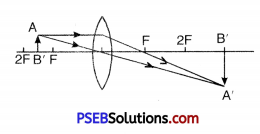3. Virtual image can not be obtained on a screen Example : (a) If the object is between the focal point of the concave mirror and the object, then the image is large, upright and virtual.In the convex mirror for each position of the object, the image obtained is virtual, erect and small. (b) If the object is between the focal point of the convex lens and the lens, then image is virtual, erect and large.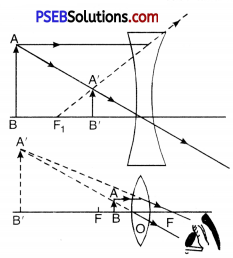Question (ii)
A person is standing 5m away from a plane mirror. How far will his image be:
(a) from the mirror
(b) from the person himself ?
Solution:
We know that the image formed in a plane mirror is at the same distance behind the mirror is as the object is in front of the mirror. So,
(a) Distance of image from the mirror = Distance of person from the mirror
= 2 meters

(b) Distance of the image from the person = Distance of the person from the plane mirror + Image distance from the mirror. = 2 meters + 2 meters
∴ Distance of the image from the person = 4 meters

Question (iii)
Give two uses of concave mirrors.
Uses of concave mirrors:

• Concave mirror is used as a having mirror, as the concave mirror produces a large and erect image when the mirror is placed close to the face.
• Concave mirrors are mostly used on the headlights of vehicles. Here the bulb (light source) is placed on the principal focus of the concave mirror.

Question (iv)
State two differences between convex lens and concave lens.
Difference between convex lens and concave lens:

 Convex lens Concave lens (1) It is thick in the middle and thin at the edges. (1) It is thin in the middle and thick at the edges. (2) It generally forms a large image of the object. (2) It forms a small and virtual image of the object.

Question (v)
Of how many colours is white light made of ? Name them.
White light consists of seven colours. Their names are:

1. Violet,
2. Purple (Indigo),
3. Blue,
4. Green.
5. Yellow,
6. Orange and
7. Red.

Question (vi)
Ravi is observing his image in a plane mirror. The distance between the mirror and his image is 6 m. If he moves 2 m towards the mirror, then the distance between Ravi and his image will be:
Given, the distance between the plane mirror and the image = 6 meters
We know, the distance between the object (Ravi) and the mirror = Distance between the mirror and the image
Distance between Ravi and mirror = 6 meters (Given.)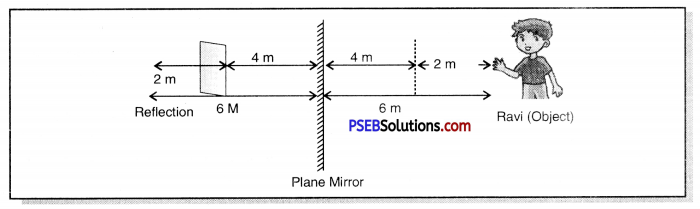When he moves 2 meters forward then the distance between Ravi and mirror will be
= 6 meters – 2 meters
= 4 meters.
Now,
Distance between Ravi and his image = Distance between Ravi and Plane mirror + Distance between plane mirror and image
= 4 meters + 4 meters
= 8 meters

Question (i)
State and explain the characteristics of the image formed by a plane mirror.
Characteristics of the image formed by the plane mirror:

• The image of the burning candle (object) is seen behind the mirror at the same distance. Therefore the image formed by a plane mirror is always formed as far behind the mirror as the objects is in front of it.
• Since the image of the candle behind the mirror cannot be obtained on the screen, therefore, The image formed by the mirror is always virtual.
• When we place the candle upright the image formed is also upwards. This shows that the image formed by a plane mirror is erect.
• When we compare the size of the candle and its image formed in a plane mirror, they are of the same size. This shows the size of the object and the size image formed by the plane mirror is equal.
• The distance between the candle and the mirror is equal to the distance between the image of the candle and the minor.
• The image formed by a plane mirror is laterally inverted. That is, the left side of the object appears to the right side of the image and the right side of the object is to the left side of the image.

Question (ii)
What is meant by dispersion of the light ? Explain by using a Prism. Which natural phenomenon is associated with dispersion of light ?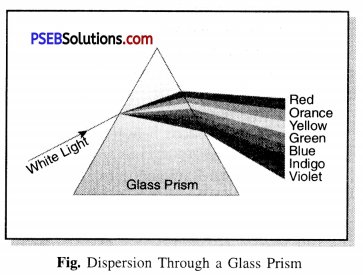Dispersion of Light.
It is the phenomena of spliting the white light into its seven colours. The process of its separation into seven colors is characterized by a white ray of light passing through the prism as shown in the figure.

Place a white screen on the other side of prism. You will see that the white light will be splited in seven colors. These colors form a band of the seven colors received on the screen called the spectrum. Due to the different wavelengths of the seven colors, these seven colors diverge at different angles. These seven colors are: (1) Violet, (2) Indigo, (3) Blue, (4) Green, (5) Yellow, (6) Orange, (7) Red.

We can remember these names with the word VIBGYOR formed from their first letters. You may have seen rainbows forming in the sky after rain. It causes the rays of the sun’s white light to be dispersed from the water droplets hanging in the atmosphere. Here the water droplets act like a prism and split up the white sunlight into its seven colours.PSEB Solutions for Class 7 Science Light Important Questions and Answers

1. Fill in the Blanks:

(i) An image that cannot be obtained on a screen is called …………………..
virtual image

(ii) Image formed by a convex …………………. is always virtual and smaller in size.
mirror

(iii) An image formed by a ………………….. mirror is always of same size as that of an object.
plane

(iv) An image which can be obtained on a screen is called a ………………. image
real

(v) An image formed by a …………………. lens cannot be obtained on a screen.
concave

2. Match the Column ‘A’ with Column ‘B’:

 Column ‘A’ Column ‘B’ (i) Convex lens (a) Rainbow (ii) Real, inverted and equal image (b) Concave mirror (iii) Alphabetical process (c) Magnifying glass (iv) Virtual image (d) Convex lens (v) The reflecting surface is inward (e) Cannot be obtained on screen

 Column ‘A’ Column ‘B’ (i) Convex lens (c) Magnifying glass (ii) Real, inverted and equal image (d) Convex lens (iii) Alphabetical process (a) Rainbow (iv) Virtual image (e) Cannot be obtained on screen (v) The reflecting surface is inward (b) Concave mirror.

Question (i)
Light travels in:
(a) Straight lines
(b) Curved lines
(c) Circles
(d) None of the above.
(a) Straight lines.Question (ii)
Erect, virtual and diminished image is formed in:
(a) plane mirror
(b) concave mirror convexo.
(c) convex mirror
(d) concave mirror
(c) convex mirror.

Question (iii)
To see an object it is necessary:
(a) Source of light
(b) Source of light and an object
(c) Source of light, object and an eye
(d) None of these.
(c) Source of light, object and an eye.

Question (iv)
After passing through a prism, light is split up into:
(a) two colours
(b) five colours
(c) seven colours
(d) six colours.
(c) seven colours.

Question (v)
To see magnified view of teeth, dentists use:
(a) plane mirror
(b) concave mirror
(c) convex mirror and convex mirrors.
(d) combination of concave
(b) concave mirror

Question (vi)
Which mirror is used to get magnified and real image ?
(a) convex mirror
(b) plane mirror
(c) concave mirror
(d) none of the above.
(c) concave mirror.

Question (vii)
Convex lens is:
(a) thick at the centre and thin at the edges
(b) thick at the edges and thin at the centre
(c) uniformly thick
(d) irregularly thick.
(a) thick at the centre and thin at the edges.

Question (viii)
When Newton disc is rotated fast which colour is seen ?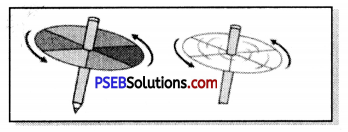(a) Black
(b) White
(c) Blue
(d) Yellow.
(b) White.Question 1.
Give one property of light.
Light travels in a straight line.

Question 2.
Which surface acts as a mirror ?
Any smooth and polished surface.

Question 3.
If you are standing in front of a plane mirror and observing your own image what is the relation
Distance of the object from the mirror = Distance of the Image from the mirror.

Question 4.
What are spherical mirrors ?
Spherical Mirrors. Spherical mirrors are the part of hollow spherical reflecting surface of which the mirror is a part. The spherical mirrors are of two types:

1. Concave mirrors
2. Convex mirrors.

Question 5.
One wants to get an enlarged image of an object in a mirror. What type of mirror should one use ?
Concave mirror.

Question 6.
What type of mirror is used in automobiles for the benefit of drivers ?
Convex mirror.

Question 7.
What type of mirrors are used in search-lights and head lights of cars ?
Concave mirror.Question 8.
Name the mirror in which the image of object is always diminished and virtual.
Convex mirror.

Question 9.
Name the kind of mirror which produces a diminished real image.
Concave mirror.

Question 10.
Write a note on convex lens.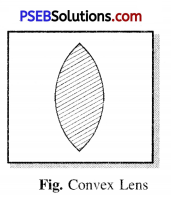Convex lens.
A convex lens is thick in the centre and thin at the edges. This lens has the capability to converge a beam of light. So this lens is also called a converging lens.

Question 11.
What is a concave lens ?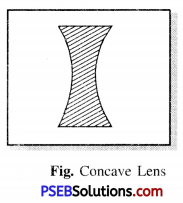Concave lens.
A concave lens is thin at the centre and thick at the edges. This lens has a capacity to diverge a beam of light. So this lens is also called a diverging lens.

Question 12.
Name the seven constituent colors of light.
Violet, Indigo, Blue, Green, Yellow, Orange, Red.

Question 13.
Describe a natural phenomenon in which seven colors of light are displayed.
View of the rainbow after rains.

Question 1.
Why should not we see towards sun through a convex lens ?
A convex lens is a converging lens. It converges a beam of parallel rays coming from the Sun at the focus. If a piece of paper or cloth is placed at the focus of a convex lens from the sun, these catch fire. So, if we see towards the sun through a convex lens, large amount of heat will be produced which is very harmful for the eyes. Hence we should not see the sun through a convex lens.

Question 2.
What is a virtual image ? Give one situation where a virtual image is formed.
Virtual image. An image which cannot be obtained on a screen, is called a virtual image.
Image formed in a plane mirror is always virtual.
Image in a concave mirror is virtual when object is placed very close to the mirror.
Image formed in a convex mirror is always virtual.Question 3.
State two differences between a convex and a concave lens.
Differences between a Convex and a Concave lens:

 Convex lens Concave lens 1. It is thick at the centre and thin at the edges.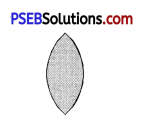It is thin at the centre and thick at the edges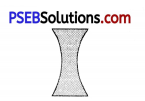2. The image is generally formed on the other side of the lens (except when the object is between F and optical centre). The image is formed on the same side of the object. 3. It converges a beam of light to a It diverges a beam of light as coming from a point.

Question 4.
Give one use each of a concave and a convex mirror.
Use of concave mirror. It is used as reflector in a searchlight or car head-light.
Use of convex mirror. It is used in cars and other vehicles as a rear view mirror.

Question 5.
Which type of mirror can form a real image ?
A concave mirror forms a real image.

Question 6.
Which type of lens forms always a virtual image ?
Concave lens.

Question 7.
Give the conditions necessary for seeing an object.
Conditions for seeing an object. To see an object the following three conditions are required to be satisfied:

1. Source of light to make the object visible.
2. The object.
3. Eye.

Question 8.
What are the uses of mirrors ?
Uses of Mirrors. We use mirrors in our daily life for one or the other purpose.
(i) Plane Mirror. Plane mirrors are used in the barber’s shops and in homes as looking glass. It is also used in periscope and other optical instruments.

(ii) Concave mirror.

1. It is used as reflector in a search light or in a car head light.
2. It is used in the solar cookers to converge the radiations coming from the sun.

(iii) Convex mirror.

1. It is used in the cars and other vehicles as a rear view mirror to have look at the vehicles coming behind.
2. It is used in amusement parks for showing strange looking images of people who stand in front of them.

Question 1.
Give an experiment to show that white light is made up of seven colours.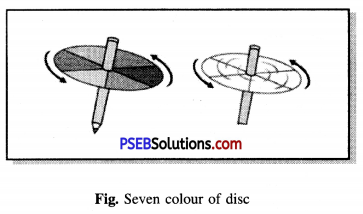White light is a mixture of seven colours. To prove that white light is a mixture of seven colours, following activity is performed.

Make a small top with rotating disc. Paint the upper surface of disc with sectorial area proportionately to the seven colours of rainbow. Now rotate the disc when a white colour will appear on the disc.
Light helps us to see the objects around us.Light PSEB 7th Class Science Notes

• A ray coming from source of light falls on the object and after reflection enters our eyes so that we see the objects.
• Light always travels in a straight line.
• The reflection from the surface of the object must be the same to see the image.
• After hitting a surface, the light returns to the same medium in a certain direction. The process of coming back of light is called reflection of light.
• The ray of light that strikes an object is called an incidental ray and the ray of light which after hitting an object returns to the same medium a particular direction in, is called a reflected ray.
• The angle between the incident ray and the perpendicular drawn at the point of incidence is called the Angle of Incidence.
• The angle between the reflected ray and the perpendicular drawn at the point of reflection is called angle of reflection.
• The angle of incidence and the angle of reflection are always equal. This is called the law of reflection.
• The image formed on screen is called Real image.
• The image that cannot be obtained on the screen even if the reflected rays appear to meet
is called Virtual image.
• The image formed by a plane mirror is always formed behind the mirror. This image is virtual is erect and of the same size as that of the object.
• The image formed by a plane mirror is at the same distance behind the mirror as the object is placed in front of the mirror.
• The image formed by a plane mirror is laterally inverted i.e. the left side of the object becomes to the right of the image and the right side of the object is seen to be left of the image.
• A concave mirror is a spherical mirror with a reflecting surface facing inwards.
• A convex mirror is a spherical mirror with a reflecting surface facing outwards.
• The rays of light coming from a distant object are considered to be parallel to each other and at the point at which they actually meet after being reflected from the mirror or appear to meet, is called the focal point of the mirror.
• For a concave mirror, a virtual, erect and large image is formed only when the object is placed between the principal focus and the mirror.
• For a convex mirror the image formed is erect, virtual and smaller in size than the size of the object.
• Lens is a piece of transparent medium surrounded by two surfaces. The two main types of lenses are – (1) Convex lens and (2) Concave lense.
• The convex lens is thicker in the middle and thinr at the edges.
• The concave lens is thin in the middle than at the edges.
• Convex lens is also called Convergent lens whereas Concave lens is called Divergent lens.
• The convex lens can be used to see small objects. Hence it is also called magnifying glass or reading glass.
• On allowing white light to pass through a prism, it splits up into seven colours, which means white light is composed of seven colours.
• The point at which the rays of light coming from a distant object (such as the sun) meet or appear to meet after suffereing refraction from the lens is called the focal point of the lens.
• When ray of light enters from one medium to another transparent medium, it changes its path. The prenomenon of light changing its path is called refraction of light.
• Reflection of light: When light travelling in a straight line strikes the surface a mirror or a polished opaque surface, it changes its direction and returns to the same medium. The process of coming back of light is called reflection of light.
• Incidental ray: A ray of light falling on the smooth and polished surface is called incident ray.
• Reflected Ray: A ray of light after striking a mirror changes its direction and returns to the same medium, is called a reflected ray.
• Angle of incidence: The angle between the incident ray and the perpendicular drawn at the point of incidence is called angle of incidence.
• Angle of reflection: The angle formed between reflected ray and perpendicular drawn at the point of incidence is called angle of reflection.
• Point of incidence: The point at which the incident ray strikes the surface of the mirror, is called the point of incidence.
• Perpendicular: The normal or perpendicular line drawn from point of incidence.
• Real Image: When the light coming from an object after reflection meet at a point forming an image then such image is called a real image.
• Virtual reflection: When the rays of light only appear to meet at a point after reflection from a mirror then that point is called virtual image. Virtual image cannot be obtained on the screen.
• Spherical Mirror: A mirror whose reflecting surface is a part of a hollow spherical surface.
• Concave mirror: If the inner surface of spherical mirror is reflecting, it is called concave mirror.
• Convex mirror: If the outer surface of the spherical mirror is reflecting, it is called convex mirror.
• Light Reflection: The bouncing back of light after when light strikes a smooth surface.
• Refraction of Light: When a ray of light enter from one transparent medium to another transparent medium, the ray of light change its path. The phenomenon of change of path by light is called refraction.
• Convex lens: This is a piece of transparent shell that is thicker in the middle than the edges. It is also called converging lens.
• Concave lens: This is a piece of transparent medium that is thin in the middle and thick at the edges. This lens is also called diverging lens.
• Focus: The point on the principal axis where the parallel rays of light meet or appear to meet after passing through the lens is called the focus.
• Focal length: The distance between the principal focus and the center of the lens is called the focal length of the lens.
• Splitting of light: The process of splitting by when white light passes through a transparent medium (such as a prism of glass) of white light into seven colours is called the splitting of light.
• Spectrum: It is a band of seven colours obtained after passing white light through the prism. These colors from top to bottom are Violet, Indigo, Blue, Green, Yellow, Orange and Red.

Punjab State Board PSEB 7th Class Science Book Solutions Chapter 15 Light Textbook Exercise Questions and Answers.